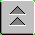[Index]

# Interferometry

LASER INTERFEROMETRY

Lasers can be used to measure very small changes in distances. This measurement in called interferometry. The only suitable source for interferometric measurements is a laser. The reason will be apparent when you see how it works. The characteristic of laser light that makes it work is coherence, meaning the light has only one frequency (or a very narrow range of frequencies). Here's how it works:

Interferometry is just the measurement of phase differences between transmitted and received laser light. For example:

When the object moves, the change in position causes a change in the phase difference at the receiver. This can be calculated simply:

If the object moves by a distance = Dx, the path travelled by the light changes by 2Dx (it is a two- way trip)

The phase change caused by a path length change of 2Dx is: Df = 2p/l (2Dx).

Here is the advantage of interferometry: Small changes in distance cause changes in the phase difference. How small? You can see quickly that distances need only be a fraction of the wavelength of the light. Suppose you can measure a phase difference change of 1/10 th of a cycle (36o). That is equivalent to changing the path length by 1/10 th of a wavelength. The change in distance to the object need only change by half of that, so 1/20 th of a wavelength. For your standard He-Ne laser (0.6328 m), that means you can detect changes in position by 1/20 x 0.6328 = 0.03 nm!